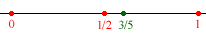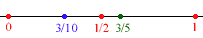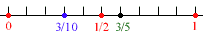Quandaries and Queries Fourth Grade Question: I would like to know is 3/5 CLOSEST to 0, 1/2 or 1. And can 3/10 go either way as to be closest to 0 or 1/2. Please help. I'm a fourth grader and I'm having an argument with my teacher. Hi, If you draw an acurate diagram it is easy to see that  3/5 is closer to 1/2 than it is to either 0 or 1.If you now put 3/10 on the same diagram you can see that it is closer to 1/2 than it is to 1.To draw an acurate diagram I divided the line segment from 0 to 1 into 10 equal pieces. Then I could see where to put all your fractions and "measure" the distances between them by counting the small intervals..For example the distance between 3/5 and 1/2 is one tenth( 1/10) and the distance between 3/10 and 3/5 is 3/10. When measuring the distance between two points to determine how close they are, we can measure in either direction. Cheers, Penny Go to Math Central